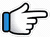# Muskurane Ki Wajah Tum Ho – Keyboard Notes## Muskurane Ki Wajah Tum Ho Keyboard NotesFor Muskurane Ki Wajah Tum Ho Notes as Western or Indian Notations, Click –

Western –
Indian –

## Keyboard Notes for Muskurane Ki Wajah Tum Ho – Indian Notation (srg mpdn)

n~ R* r*R*n       n     R*r*
Musku_rane      ki    wajah

nM*      g*~ r*R*nR*d~
tum       ho~~~
Or
nM*      g*~ r*R*nR*nd
nM*      g*~ r*R*nd~~

d  nR*nd           d     nR*n
Gun_gunane    ki    wajah

dg*     r*~ R*ndp
tum    ho
Or
dg*     r*ndpndp

R*n       Nn        R*
Jiya       jaaye      na,

Nn        R*     Nn       R*~
jaaye    na,    jaaye   na

N     N     R*~ n      n~~
O     re     piya       re

n     R*     r*R*n
O    re       lamhe,

n       R*r*R*
tu      kahin

nM*      g*~ r*R*nd~~
mat       jaa~~
Or
nM*       g*~ r*R*nR*d~
nM*       g*~ r*R*nR*nd

d~     nR*~   nd
Ho    sake  _   toh
Or
d~    nR*n      d

dn          R*n
umr       bhar

dg*         r*~ R*ndp
tham      jaa
Or
dg*         r*ndpndp

R*n      Nn      R*
Jiya     jaaye    na,
Or
nn        Nn      R*

Nn       R*     Nn        R*
jaaye   na,    jaaye    na

N     N      R*~ n      n
O     re     piya        re

R*n      Nn      R*
Jiya      jaaye    na,

Nn        R*     Nn      R*
jaaye    na,    jaaye    na

M*     g*      p*~M*         r*~~  R*g*~  R*nd~
O        re       piya           re~~~
Or
M*     g*      p*~M*        r*~~  g*~  R*nd~
M*     g*      p*~M*        r*~~ r*g*r*R*

R*~ n~       n~~
Piyaa         re~~~
Or
R*r*           nr*~ R*~ nn

M*g*M*    r*~R*~     r*~ r*g*M*
Dhoop       aaye          toh~~,

M*g*M*      r*     r*g*r*R*
chhaanv    tum    laana
Or
M*g*M*    r*     r*R*R*

dR*g*~ g*        g*
Khwahishon    ki
Or
dR*M*g*           g*

dR*g*~ g*        g*
baarishon      mein

r*M*g*       r*R*      nR*r*~~
Bheeg         sang      jaana

R*n       Nn      R*
Jiya      jaaye    na,
Or
nn        Nn      R*

Nn        R*      Nn        R*
jaaye    na,    jaaye    na

N     N      R*~ n     n
O     re     piya        re

R*n     Nn        R*
Jiya     jaaye    na,

Nn       R*     Nn        R*
jaaye   na,    jaaye    na

M*     g*      p*~M*        r*~~  R*g*~  R*nd~
O       re       piya             re~~~
Or
M*     g*      p*~M*        r*~~  g*~  R*nd~
M*     g*      p*~M*        r*~~ r*g*r*R*

R*~ n~       n~~
Piyaa            re~~~
Or
R*r*        nr*~ R*~ nn

Will be Updated:
Jo    miley    usme
kaat    lenge    hum
Thodi    khushiyaan,
thode    aansu
baant    lenge    hum
Muskurane    ki    wajah
tum    ho
Gungunane    ki    wajah
tum    ho